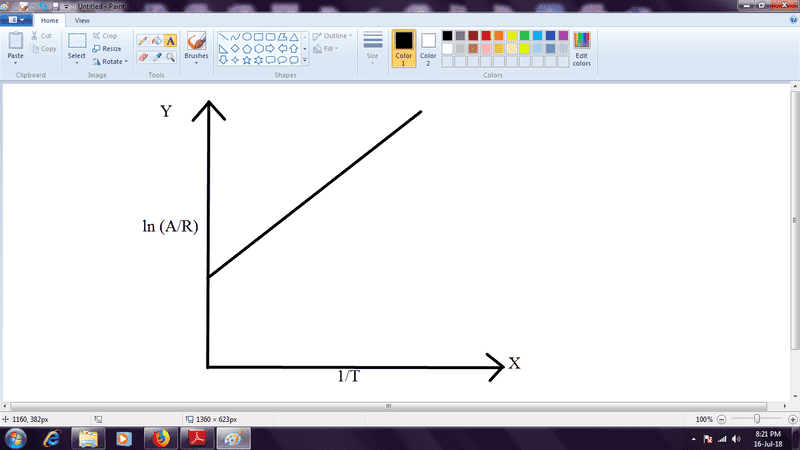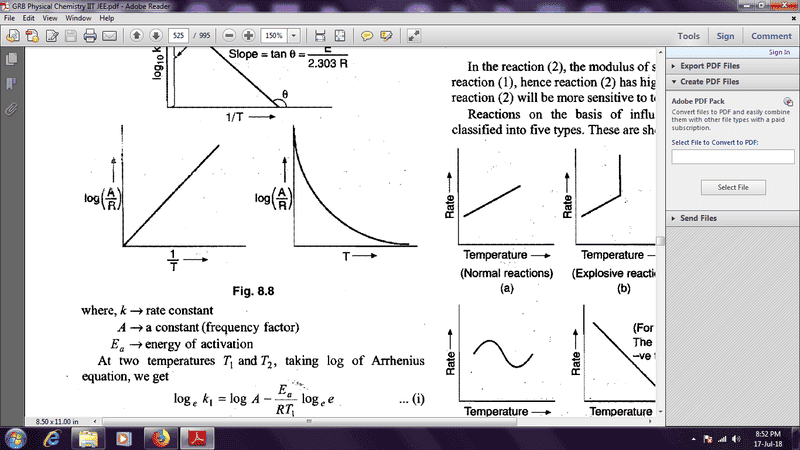# Graph of ln(A/R) vs 1/T

In summary, this book claims that the graph of ln(k/R) vs 1/T should be a straight line parallel to the x-axis, with a constant y value of ln(k/R) + E/RT. However, the next graph on the page is definitely wrong, and the one for log in which base 10 is not mentioned is also wrong.f

## Homework Statement

Draw a probable graph of ln (A/R) vs 1/T.

## Homework Equations

Arrhenius equation: k= Ae-E/RT

## The Attempt at a Solution

Taking natural log on both sides of arrhenius equation :
ln k = ln A - E/RT
ln k - ln R = ln A - E/RT - ln R (subtracting ln R from both sides)
ln (k/R) = ln (A/R) - E/RT
rearranging terms and comparing it with y=mx + c,
c= ln (k/R) and m = E/RAccording to me the graph should be like this. In the answers, the graph is a similar one, except that there is no intercept given. I am confused. Any help is appreciated.

#### Attachments

Are you sure you've got your terms right? A in the Arrhenius equation is a constant independent of T. And you can't write ln(k/R) = c because k certainly varies with T.

@mjc123
ln(k/R)=c, by 'c' i don't mean constant, i mean the y-intercept.
Regarding the terms, yes i am sure. Though A and R are both independent of T, the graph could also have been straight line parallel to x axis. Any help is appreciated.

The graph must be a straight line parallel to the x axis, with a constant y value of ln(k/R) + E/RT. But it seems a strange graph to plot anyway.
BTW, c in y = mx + c is a constant. It's not just the value of the y-intercept, it's telling you that the only x-dependence of y is in the term mx.

Ok, got it. Should i send a screenshot of that page of the book? (for confirmation)

If it has the text of the question, that would help.

The question is : Which of the following graphs is correct ?
the answer : fig 8.8 first from leftEven the very next graph is wrong.
log in which base 10 is not mentioned is natural log according to this book.
There were only 3 options, the other two are absolutely wrong, simple arrhenius plots.

#### Attachments

That's definitely wrong. A is a constant in the Arrhenius expression. If A varied with temperature, you couldn't get E from the slope of the ln k vs. 1/T plot.

Got it, thanks.